# Measures of Variability : Interquartile Range

The interquartile range is the difference between quartile 3 (upper quartile) and quartile 1 (lower quartile). It is one way of describing the spread of the data.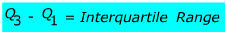Let's look at a couple of examples.

Find the interquartile range of the following data.

Example 1:

1, 7, 0, 7, 2, 6, 3, 6, 0, 7, 8

First make sure that it is in order from least to greatest.

0, 0, 1, 2, 3, 6, 6, 7, 7, 7, 8

Find the median:
0, 0, 1, 2, 3, 6, 6, 7, 7, 7, 8  6 is the median
(middle number and Q2)

Find the middle of the
0, 0, 1, 2, 3, 6, 6, 7, 7, 7, 8  Q1 = 1
first half of the numbers

Find the middle of the
0, 0, 1, 2, 3, 6, 6, 7, 7, 7, 8  Q3= 7
Second half of the numbersExample 2:
10, 1, 7, 5, 1, 8, 5, 4, 6, 5, 9, 12

Put in order from least to greatest

1, 1, 4, 5, 5, 5, 6, 7, 8, 9, 10, 12

find the average

Find the median   1, 1, 4, 5, 5, 5,6, 7, 8, 9, 10, 12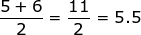Find Q1 the median of the lower half

1, 1, 4,5, 5, 5,6, 7, 8, 9, 10, 12Find Q3the median of the upper half

1, 1, 4,5, 5, 5,6, 7, 8,9, 10, 12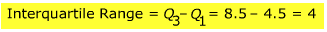Let's look at an example when given a box and whisker plot.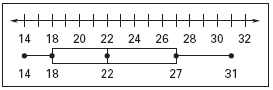Min         Q1           Med         Q3         Max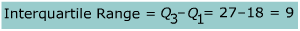A quick review: to find the interquartile range you will put the data in order from least to greatest then find the median. Once you have found the median Q1is the median of the first half of the data and Q3is the median of the second half of the data.

 Related Links: Math algebra Measures of Variability : Range Parts of An Expression

To link to this Measures of Variability : Interquartile Range page, copy the following code to your site: